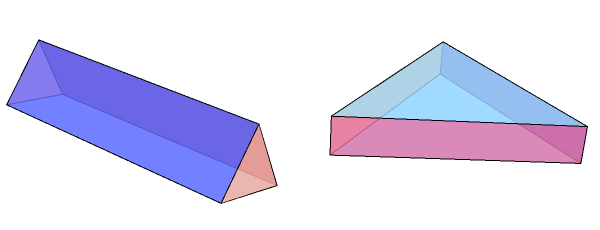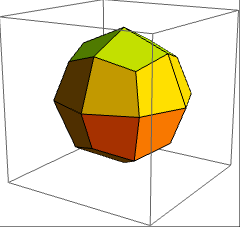# Fair dice

(I’m testing out the functionality of the cp4space Facebook page. Hopefully, this post should be automatically published there.)

In most familiar board games, cubic dice are used on the basis that each face is equally likely to face upwards. In prehistoric times, tetrahedral bones were used, and other polyhedral dice are prevalent nowadays. It is not too difficult to see that all regular, and indeed all face-transitive, dice are all trivially unbiased.

What about dice which are not face-transitive? Brent Meeker gave a non-constructive example of such a die. Consider an equilateral triangular prism. By choosing the proportions, it is possible to make the triangular faces more likely than the rectangular faces, or vice-versa.By the Intermediate Value Theorem, we can find some optimal ratio that gives all faces a probability of 1/5. However, this ratio is dependent on the physical model. We’ll consider two plausible physical models here, and show that each of them give a different optimal proportion.

1. The LGS model: We have a large vat of Lyle’s Golden Syrup, into which we drop the die vertically. In this model, the fluid is sufficiently viscous that the inertia of the falling die can be ignored. Specifically, the die falls vertically until a vertex touches the base of the vat. Then, gravity causes the die to roll onto the face directly below the centroid. The probability of a face is proportional to the solid angle subtended by the face from the centroid if the projection of the centroid lands inside the face, and zero otherwise.
2. The agitated adhesive model: The die rests on the surface of a steel drum, which is covered in glue. Every second, the drum is hit by a long metal baton, causing the die to spontaneously jump into the air. Each time it lands, there is a probability (an increasing function f, proportional to the kth power of the contact area) of the die permanently sticking to the surface of the drum. As k tends to infinity, a fair die must have all faces with equal area.

With a little help from RIES, I was able to determine that the ratio of edge lengths for a fair die in the LGS model is$\dfrac{2 \sqrt{\sqrt{5}}}{\sqrt{3} (1+\sqrt{5})}$, compared with$\dfrac{\sqrt{3}}{4}$ in the limit of the agitated adhesive model.

### Pseudo-deltoidal icositetrahedron

The closest thing to a uniform polyhedron without being itself uniform is one of the Johnson solids, the elongated square gyrobicupola. Its projective dual is thus almost face-transitive without actually being face-transitive. It’s a fair die in both of the models shown above, as well as absolutely any other physical model I can imagine. It has the following properties:

• All faces are congruent;
• The incentre and centroid are well-defined and coincide;
• The inertia tensor is isotropic.

A rotating model of the pseudo-deltoidal icositetrahedron is below:Does there exist a natural physical model in which the pseudo-deltoidal icositetrahedron is not a fair die? In particular, is this the case for the simple Newtonian model?

This entry was posted in Uncategorized. Bookmark the permalink.

### 0 Responses to Fair dice

1.Andrew Carlotti says:

Is it possible that it is possible to throw a non-face transitive die in two different ways, such that both appear to be random throws but the dice is biased in a different way for each throw. (I know this is very imprecise, but I can’t see how to formalise it.)

•apgoucher says:

The triangular prism, where the edges abutting two rectangular faces are half as long as the other edges, has the property that triangles are favoured in one model and rectangles are favoured in the other.

•Andrew Carlotti says:

I suppose that could count. A clearer idea though might be throwing dice so it rolls in one of two orientations (end over end, or on its side). However, since this argument could also be applied to a standard cube die, it seems rather odd.

•apgoucher says:

Alternatively, there may be face-transitive dice with bilateral symmetry, where a left-handed throw favours half of the faces, and a right-handed throw favours the other half.

Specifically, the disdyakis dodecahedron and triacontahedron may satisfy this property:

(They’re duals of omnitruncated regular solids.)

2.Farida Flanelle says:

I’d go with the second model; I can think of far better uses for Lyle’s Golden Syrup… ;P